﻿ St. Mary's H.S. Physics - Intro to Friction Notes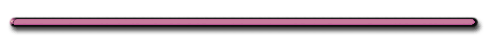Friction

D) Friction - force between 2 surfaces that opposes motionDepends only on: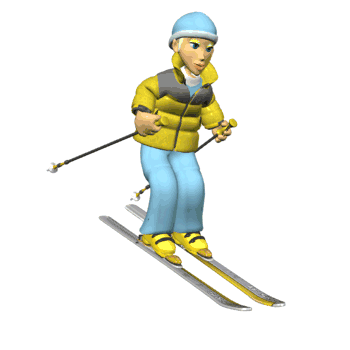1) texture of each surface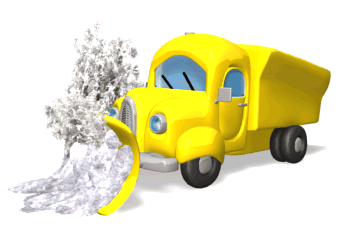What do plows have on their wheels to increase  friction?

Chains

2) force holding objects togetherWhat do race cars have mounted on their rear hood to increase  their friction?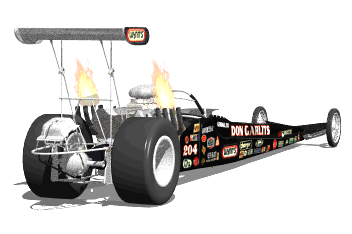Spoilers increase the force between tire and road

Two Kinds of Friction

(fs) 1) Static Friction - force of friction that must be overcome to move an object at rest.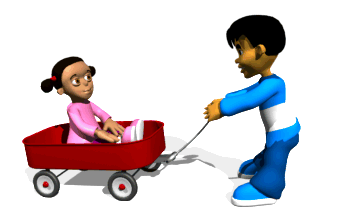(fk) 2) Kinetic Friction - force of friction that opposes a moving object.

Kinetic friction is weaker than static friction

fk < fs

Friction Equations

Static friction: fs = usFn

Kinetic friction: fk = ukFn

Friction on Ramp in terms of m, theta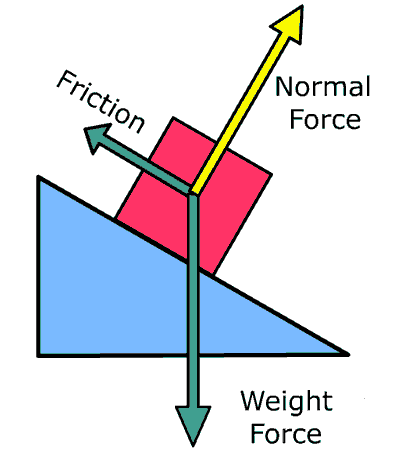f = U[mgcosq]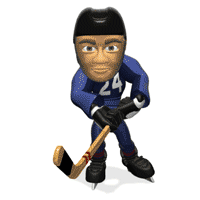ice and skate - low uk

Friction on horizontal surface

Level Force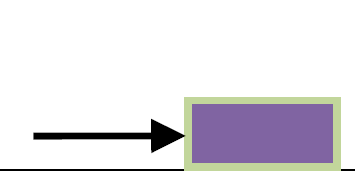fs = uFn = umg

Friction on horizontal surface

Tilted Push, F, q, m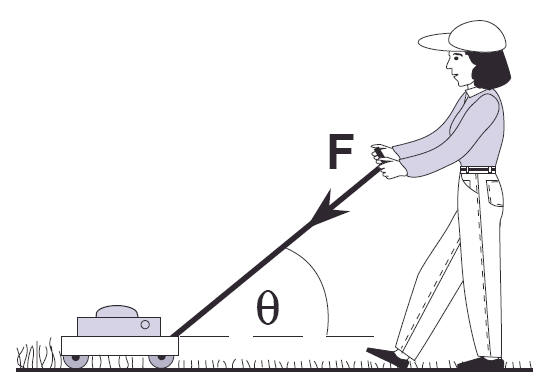fs = uFn = ?

Fn = Net downward force on lawn mower

Fn = [mg + Fsinq]

fs = u[mg + Fsinq]What if mower was pulled?

fs = u[mg - Fsinq]